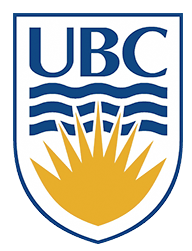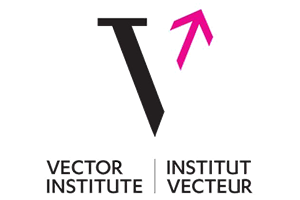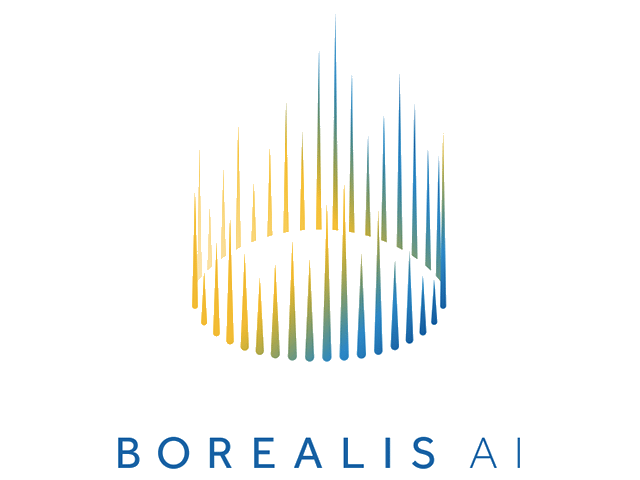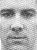# Leonid Sigal

## Associate Professor, University of British Columbia| | | | | | | |

# Contact

Dep. of Computer Science
University of British Columbia
ICCS 119
2366 Main Mall
Vancouver, B.C. V6T 1Z4
CANADA

Phone: 1-604-822-4368
Email: lsigal at cs.ubc.ca

Due: At the end of the day 11:59pm, Friday, Februrary 8, 2019.

The purpose of this assignment is to implement and understand the use of normalized cross correlation for object detection in a scaled representation.

Again, there are different ways to import libraries/modules into Python. Styles and practices vary. For consistency (and to make life easier for the markers) you are required to import modules for this assignment exactly as follows:

```	    ```
from PIL import Image, ImageDraw
import numpy as np
import math
from scipy import signal
import ncc
```
```

## The assignment

1. The zip file containing ncc.py, sample images and a face template is hw2.zip. Download this file to your directory and unzip with the command

```        ```
unzip hw2.zip
```
```

The face template we will use is in the file face_detection_template.jpg and is shown below.2. `(2 points)`

The first task is to build a scaled representation of the input image. Create a routine pyramid = MakePyramid(image, minsize) that creates a pyramid for an image. It returns a list including the original PIL image followed by all the PIL of images of reduced size, using a scale factor of 0.75 from one level to the next. The pyramid should stop when any further reduction in size will make a dimension of the image smaller than minsize. If im is a PIL image, you can use the Python function im.resize((int(x*0.75),int(y*0.75)), Image.BICUBIC) to reduce the image size at each step. Note: Sub-sampling here uses bicubic interpolation to avoid aliasing problems.

3. `(3 points)`

In order to check your pyramid, write a routine ShowPyramid(pyramid) that will join all the images in the pyramid into one horizontal image and display this result with imshow.

You can combine multiple images into a single image by first creating a blank image of the right size image = Image.new("L", (width, height)) and then pasting existing images into it image.paste(im,(offset_x,offset_y)). Since you will be handing in a PDF version of your pyramids, feel free to initialize unused space with white (rather than black).This will make it look cleaner in the PDF.

4. `(10 points)`To match a template to a pyramid, write a function FindTemplate(pyramid, template, threshold) that will find and mark all locations in the pyramid at which the normalized cross correlation (NCC) of the template with the image is above the threshold. The goal is to achieve results similar to those on the right, although they will differ depending on the threshold you choose.

Since NCC is an expensive operation and since its cost depends heavily on the size of the template, you should use the minimum possible template size. Try reducing the width to 15 pixels using imresize with bicubic interpolation. Define a constant for template width so that it is easy to experiment with other sizes.

As you saw in Assignment 2, the Scipy Signal Processing toolbox has a function signal.correlate2d. But, this is not normalized cross-correlation. NCC needs to normalize its two inputs correctly: the template (once, since it doesn't change), and each window in the image at which the template is positioned (which, of course, changes with each position). Normalization adjusts the template and each window to have zero mean and unit magnitude.

You can compute the NCC using the function normxcorr2D provided. If image is a PIL image and template is the PIL template, then ncc.normxcorr2D(image, template) returns the array of cross-correlation coefficients, in the range -1.0 to 1.0. The returned array is the same size as image. The function, normxcorr2D, makes use of signal.correlate2d. It is not a particularly efficient implementation, but it is sufficient for our purposes. ASIDE: For an efficient implementation of NCC, one would generally link to external (non-Python) libraries, like OpenCV. For a general discussion of NCC implementation issues, see J.P. Lewis, Fast Normalized Cross-Correlation.

For each pixel at which the normalized correlation result is above the threshold, draw lines (forming a rectangle) to mark the boundary of the template at that location. All lines should be shown overlayed on the original image (the first image in the pyramid), which means that template matches at other scales will need to be adjusted by the right scale factor (i.e., by the right power of 0.75).

To draw lines in a PIL image, use the routine draw.line in the ImageDraw module. For example, if im is a PIL image,

```        ```
draw = ImageDraw.Draw(im)
draw.line((x1,y1,x2,y2),fill="red",width=2)
del draw
```
```
draws a red line of width 2 in im from (x1,y1) to (x2,y2). Of course, if you really want the line to be red, im must support colour. If necessary, you can achieve this with im = im.convert('RGB').

Since the face template was extracted from one of the faces in the judybats image, it will have a strong correlation with that location, which should help to make debugging the location output easier.

5. `(5 points)`

Once your code is working, adjust the threshold to achieve close to an equal error rate on the six given images (judybats, students, tree, family, fans, sports) considered together. An equal error rate is where the number of non-faces seen as faces (false positives) equals the number of missed faces (false negatives). You can do this by just trying different thresholds and counting the number of non-faces seen as faces and the number of missed faces.

6. `(5 points)`

For question 5, what is the recall rate of the program on each image? The recall rate is defined here: Precision and recall. Explain why the NCC method has a very low recall rate on some images.

## Deliverables

You will hand in your assignment ellectronically through Canvas. You should hand in two files, a file containig your code (i.e.,copies of your functions in a single  *.py file). These must have sufficient comments for others to easily use and understand the code. You will lose marks for insufficient or unclear comments or poorly designed code. In addition, hand in a single PDF document showing:

• scripts (i.e., records of your interactions with the Python shell) showing the specified tests of your functions
• an image showing the result of ShowPryramid (for one of the test images)
• your final selected threshold
• images with the face detections for your final selected threshold for all six images

The PDF file has to be organized and easily readible / accessible.